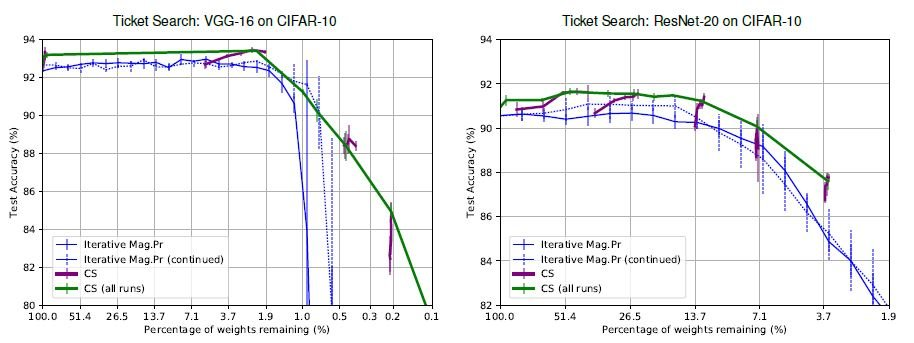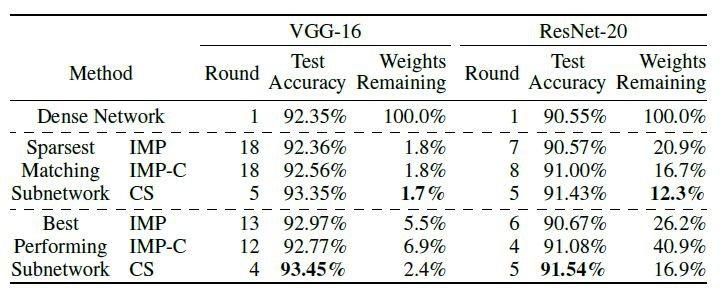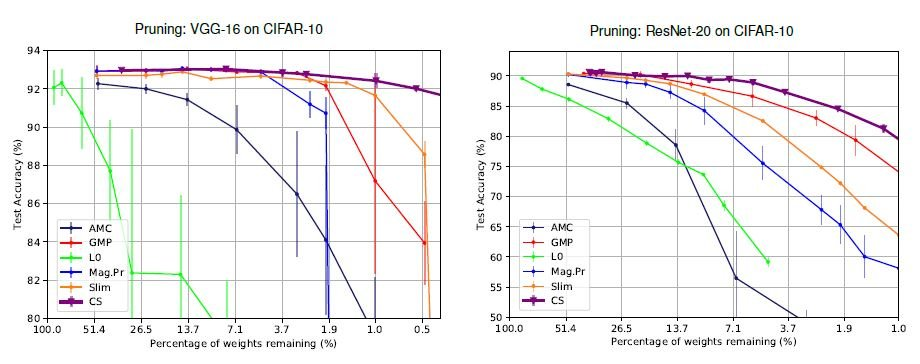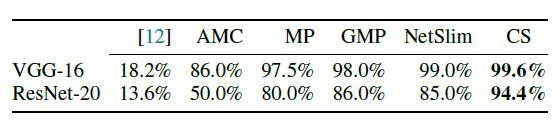# Catch up on the latest AI articles# Don't Need A Big Model For Pruning? Automatically Find Sparse Networks!

3 main points
✔️ A new Pruning method based on a new approximation of $l_0$ regularization
✔️ Higher performance than heuristic-based Pruning such as magnitude and gradient
✔️ Particularly efficient when subnetwork search is performed in parallel

Written by Pedro Savarese, Hugo Silva, Michael Maire
(Submitted on 11 Jan 2021)
Published as a conference paper at NeurIPS 2020
Subjects: Machine Learning (cs.LG); Machine Learning (stat.ML)code:.## First of all

Pruning is a technique used to reduce the weights of a deep neural network to create a lightweight model. Traditionally, branch pruning methods have been based on heuristics using network weights and gradients, but it is not clear that these metrics are truly suitable as branch pruning metrics.

In this paper, we provide optimal branch trimming using $l_0$ regularization as a non-heuristic method. We also provide a subnetwork search method with $l_0$ regularization when combined with the Lottery Ticket Hypothesis.

The experiment finds well-sparsified networks and more accurate networks in the search for subnetworks compared to the lottery hypothesis Iterative Magnitude Pruning.

## $l_0$ Pruning by Regularization

A regularization method that constrains the network weights to be zero, i.e. sparse, is $l_0$ regularization. By adding $l_0$ to the loss function, the network can be pruned appropriately during the training of the network. Also, since it is not a heuristic measure, the network will automatically find the weights to remove.

However, the $L_0$ regularization is not differentiable. Therefore. As a countermeasure, previous studies used probability distributions that generate masks of 0 and 1. However, there is a problem that the mask generated is not stable due to the bias and variance of the distribution. In addition, the subnetwork search method in the lottery hypothesis uses heuristic measures to reduce the network, but it is not clear whether these measures clearly indicate the weights to be reduced.

Therefore, in this paper, we review the formulae for $l_0$ regularization formulae are reviewed, a method to approximate the deterministic $l_0$ regularization is proposed, and the method is applied to subnetwork search to achieve SOTA.

## The proposed method (Continuous Sparsification)

In the beginning, we use $l_0$ regularization for the weight $w$ as a penalty term in minimizing the loss of the network $f$. The loss minimization problem that includes the regularization term is $$\min _{w \in \mathbb{R}^{d}} L(f(\cdot ; w))+\lambda \cdot\|w\|_{0}$$

Next, we introduce the mask $m \in\{0,1\}^{d}$. $$\min _{w \in \mathbb{R}^{d}, m \in\{0,1\}^{d}} L(f(\cdot ; m \odot w))+\lambda \cdot\|m\|_{1}$$

The mask $m$ is a boolean, so $|m\|_{0} = |m\|_{1}$. Therefore, we can change $l_0$ regularization to $l_1$ regularization. Because $m$ is a boolean, it is not differentiable by the steepest descent method. To make the penalty term differentiable, we first introduce the Heaviside function: $$\min _{w \in \mathbb{R}^{d}, s \in \mathbb{R}_{\neq 0}^{d}} L(f(\cdot ; H(s) \odot w))+\lambda \cdot\|H(s)\odot|_{1}$$

The $s$ is the new variable to be minimized, and by the Heaviside function, if $s$ is positive, the mask is 1, and if it is negative, the mask is 0. Next, we further transform the equation to be differentiable.

The expression transformed to differentiable is $$L_{\beta}(w, s):=L(f(\cdot ; \sigma(\beta s) \odot w))+\lambda \cdot\|\sigma(\beta s) \odot\|_{1}$$. is. We use the new sigmoid function $\sigma$. Also, $\beta \in[1, \infty)$ is a hyperparameter that is determined by the user during training.

When $\beta$ is close to $infty$, $\lim _{\beta \rightarrow \infty} \sigma(\beta s)=H(s)$ holds. On the other hand, when $\beta = 1$, $\sigma(\beta s)=\sigma(s)$ holds. Therefore, the following equation holds.

$$\min _{w \in \mathbb{R}^{d} \atop s \in \mathbb{R}_{\neq 0}^{d}} \lim _{\beta \rightarrow \infty} L_{\beta}(w, s)=\min _{w \in \mathbb{R}^{d} \atop s \in \mathbb{R}_{\neq 0}^{d}} L(f(\cdot ; H(s) \odot w))+\lambda \cdot\|H(s)\|_{1}$$

As a hyperparameter, $\beta$ controls the difficulty of the computation. By gradually increasing $\beta$ during learning, one may succeed in approximating the intractable problem (originating from the numerical connection).

## Experimental results

In this paper, we mainly compare the performance of subnetwork generation based on the lottery hypothesis with that of conventional pruning. First, the proposed method is compared with Iterative Magnitude Pruning, a magnitude-based pruning, in terms of its ability to generate subnetworks.The sub-networks are explored on VGG-16 and ResNet-20 trained on CIFAR-10, and the proposed method (CS) is dominant in both cases.

Iterative Mag. Pr is a size-based subnetwork search method used in the usual lottery hypothesis. Normally, when branch trimming is done in multiple rounds, the weights learned in one round are reset to the initial weights, but Iterative Mag.Pr (continued), where we did not let the thoughts reset even when the rounds were switched.

CS is the proposed method and CS (all runs) is the result of summarizing the results of each experiment and connecting each point. It is interesting to note that the accuracy of the proposed method does not decrease even when the branch trimming rate is increased. I think this effect is effective when the branch trimming is done to the limit.In the comparison of the most sparse networks, while maintaining the same performance as the baseline network, the proposed method is able to find more sparse subnetworks with 1.7 % and 12.3 % for VGG-16 and ResNet-20, respectively.

In the comparison of the best performing subnetworks, we also 93.45 % for VGG-16 and 91.54 % for ResNet-20, we find highly accurate networks. In addition, despite the good accuracy, we achieve a high branch trimming rate. Compared to IMP, the number of rounds required for training is much less. Therefore, it is possible to find subnetworks quickly. Next are the comparison results between the traditional heuristic Pruning method and the proposed method.Unlike conventional heuristic methods, there is no sudden Unlike conventional heuristics, there is no sudden drop in accuracy and Unlike conventional heuristic methods, there is no sudden drop in accuracy and the accuracy is maintained at a high level. Moreover, the conventional L0 regularization method is unstable and gives the worst results in both VGG-16 and ResNet-20.

## Summary

In this paper, we propose a new $l_0$-regularization approximation method and apply it to the subnetwork search in order to deal with the problem that the conventional $l_0$-regularization Pruning is unstable and the subnetwork search method is the only heuristic and its validity cannot be evaluated. We have achieved SOTA in both subnetwork search and pruning.

The proposed method is superior to other methods because it maintains accuracy even if the pruning rate is high. In addition, the proposed method can find subnetworks much faster than other methods if parallel computing is available.

Of particular interest is that the proposed method finds sparse and highly accurate networks in fewer rounds. In traditional Pruning, it is very hard to directly create small models, so it is common to create small models from large models. However, the proposed method finds good sub-networks early in the learning process of the original network. This is in contrast to I think this raises a question about the traditional Pruning after training.

As mentioned in this paper, the combination of transfer learning and pruning is also an interesting topic, since it has been shown that subnetworks can be transferred between similar and different tasks.

If you have any suggestions for improvement of the content of the article,
please contact the AI-SCHOLAR editorial team through the contact form.